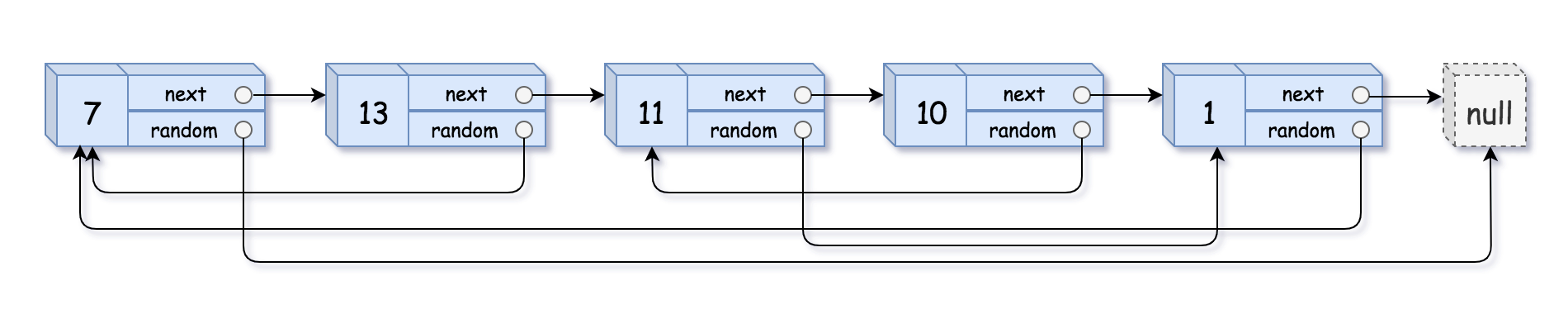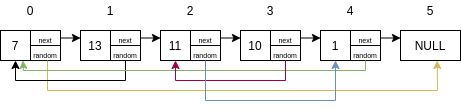## 题目描述

• val：一个表示 Node.val 的整数。
• random_index：随机指针指向的节点索引（范围从 0 到 n-1）；如果不指向任何节点，则为 null 。0 <= n <= 1000
-10000 <= Node.val <= 10000
Node.random 为空（null）或指向链表中的节点。

## 解题思路## AC代码

/*
// Definition for a Node.
class Node {
int val;
Node next;
Node random;

public Node(int val) {
this.val = val;
this.next = null;
this.random = null;
}
}
*/

class Solution {

public Node copyRandomList(Node head) {
if (head == null) {
return null;
}

Map<Node, Integer> record1 = new HashMap<>();
Map<Integer, Node> record2 = new HashMap<>();
Node root = new Node(head.val);
Node p1 = root;
Node p2 = head;
p2 = p2.next;
int i = 0, j = 0;
record2.put(j++, p1);
// 遍历旧链表，拷贝信息到新链表中
while (p2 != null) {
record1.put(p2, i++);
p1.next = new Node(p2.val);
p2 = p2.next;
p1 = p1.next;
record2.put(j++, p1);
}

p1 = root;